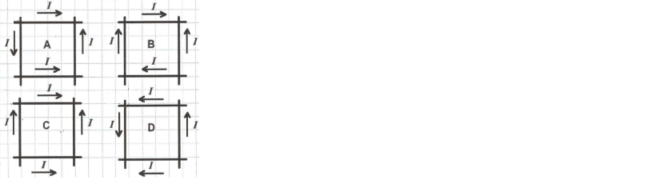Physics Practice Problems Magnetic Field Produced by Straight Currents Practice Problems Solution: The figure shows four different sets of insulated ...

🤓 Based on our data, we think this question is relevant for Professor McCormick's class at USF.

# Solution: The figure shows four different sets of insulated wires that cross each other at right angles without making electrical contact. The magnitude of the current is the same in all the wires, and the direction of current flow are as indicated. For which (if any) configuration will the magnetic field at the center of the square formed by the wires be equal to zero? A) A B) B C) C D) D E) The field is not equal to zero in any of these cases.

###### Problem

The figure shows four different sets of insulated wires that cross each other at right angles without making electrical contact. The magnitude of the current is the same in all the wires, and the direction of current flow are as indicated. For which (if any) configuration will the magnetic field at the center of the square formed by the wires be equal to zero?

A) A

B) B

C) C

D) D

E) The field is not equal to zero in any of these cases.Magnetic Field Produced by Straight Currents

Magnetic Field Produced by Straight Currents

#### Q. A long, straight wire lies along the y-axis and carries current in the positive y-direction. A positive point charge moves along the x-axis in the pos...

Solved • Thu Mar 23 2017 11:54:22 GMT-0400 (EDT)

Magnetic Field Produced by Straight Currents

#### Q. At what distance from the central axis of a long straight thin wire carrying a current of 5.0 A is the magnitude of the magnetic field due to the wire...

Solved • Tue Feb 28 2017 10:00:40 GMT-0500 (EST)

Magnetic Field Produced by Straight Currents

#### Q. Two long parallel wires carry currents of 20 A and 5 A in opposite directions. The wires are separated by 0.20 m. What is the magnitude of the magneti...

Solved • Tue Feb 28 2017 08:32:15 GMT-0500 (EST)

Magnetic Field Produced by Straight Currents

#### Q. Two long straight parallel wires carry currents as shown in the sketch. Point  A is midway between the two wires, 0.20 m from each wire. What are the ...

Solved • Wed Feb 22 2017 08:11:55 GMT-0500 (EST)